Mathematical Quilts

Some of my work...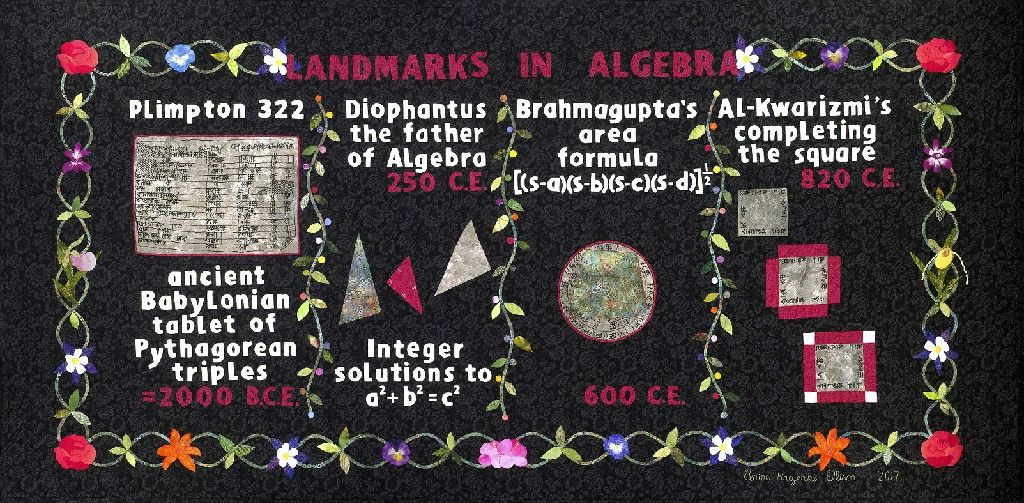Landmarks In Algebra - This four panel quilt illustrates significant contributions to the development of Algebra. The Plimpton 322 is a Babylonian Cuneiform tablet showing early knowledge of the Pythagorean Triples.  This tablet dates from approximately 2000 B. C. E. Diophantus ( b. 201 - 215 C.E., d. 285-299 C.E) is highlighted in the second panel.   Amongst his contributions to Algebra we find the importance of integer solutions to equations.   Brahmagupta's (b. 598 C.E., d. 665 C.E.) contribution to cyclic quadrilaterals is shown in the third panel.    Finally, Al-Kwarismi's ( b. 780 C. E., d.850 C. E.)
completing the square technique is shown.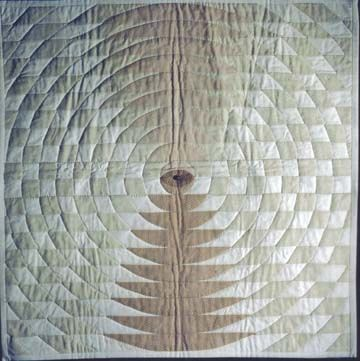Parabolas -
Hand-dyed fabric that is hand-appliqued. Menaechmus is credited
with the development of the conic sections. The time period here is 375-325 B.C.
This quilt was used during the conic section unit in Algebra II. This quilt is
owned in a private collection.Ellipse and hyperbolas -
Hand-dyed fabric that is hand-appliqued also.
Menaechmus is the mathematician involved with the discovery of the conic sections.
Menaechumus is also credited with the discovery of thse conic sections. The ellipse
and the hyperbola are confocal--that is, they share the same focal points. This quilt is owned
in a private collection.Spiraling Squares - is an appliqué quilt based on Plato’s 4th century B.C. dissection.
Plato asked the question: how can a square be cut so that two new squares are made—
the area of the two new squares will be equal to the original squares’ area. In this quilt,
each new square’s side is found by taking the diagonal of the old square. It is that simple!

A short video of this quilt can be found here.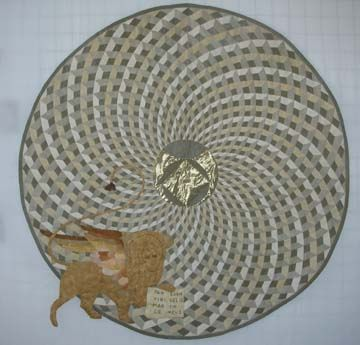St. Mark’s Basillica -
Cosmati Tiles - The original floor pattern
divided the circle into 34 sections—I divided the circle into 36 sectors, thereby making
the appliqué significantly more difficult. In the center of the quilt will be Leonardo’s
Claw—the Venetian Lion will adorn the bottom of the quilt. 1050 B.C. and Byzantine
style church with an unknown architect. A short video of this quilt can be found here.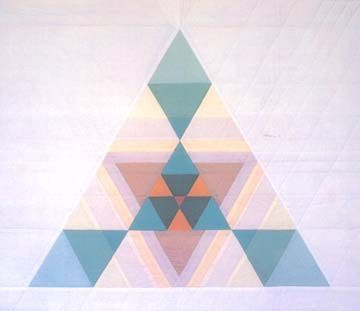Fibonacci X 3 -
Fibonacci lived from 1175-1250. The Fibonacci sequence first appeared
in print in 1202. The number pattern being: 1,1,2,3,5,8,13,21,34,55,… is attributed to
Fibonacci. As Fibonacci traveled the Mediteranean area with his merchant father,
he spread the benefits of using the Hindu-Arabic numeration system that we
use today. Thank you Fibonacci!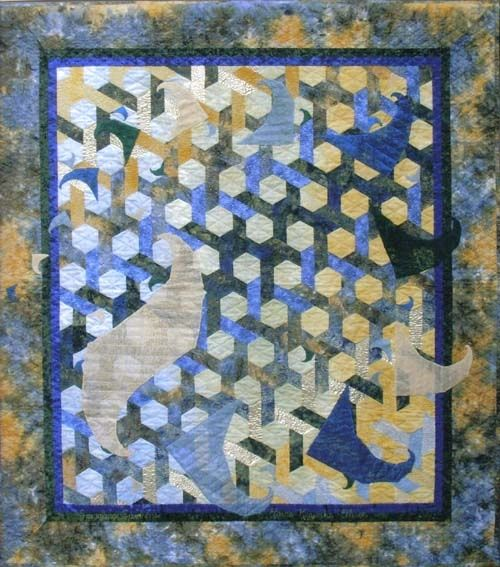Spiraling Spidrons - In the early l970's, Daniel Erdely discovered the spiraling polygon and named it a spidron.  Erdely is a Hungarian industrial designer that popularized the figure
made up of isosceles triangles and equilateral triangles.  The angles of the spidron
are 30, 60, and 90 degrees which make it a perfect triangle for study in the mathematics classroom.  An article in Science News  in 2007 interested me--so I quilted the spidron!
A short video of this quilt can be found here.Greek Cross to Square Dissection - Our Bridges Conference in Leeuwarden, Netherlands
in 2008 had a paper that caught my attention. "Making Patterns on the Surfaces of
Swing-Hinged Dissections" by Reza Sarhangi illustrated the use of two superposed tessellating grids using the same common shape. The shape forms the Greek Cross and
it also forms the squares in this quilt. Quilt completed in 2013.

A short video of this quilt can be found here.